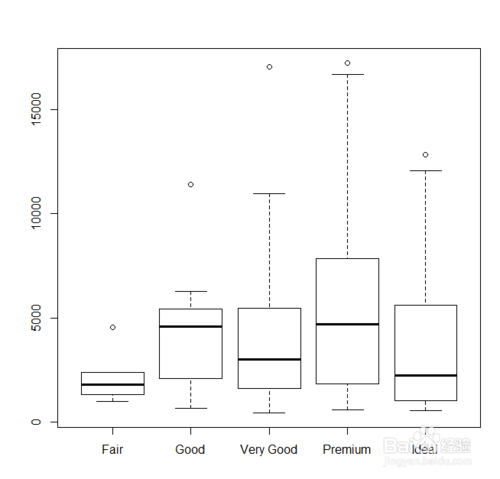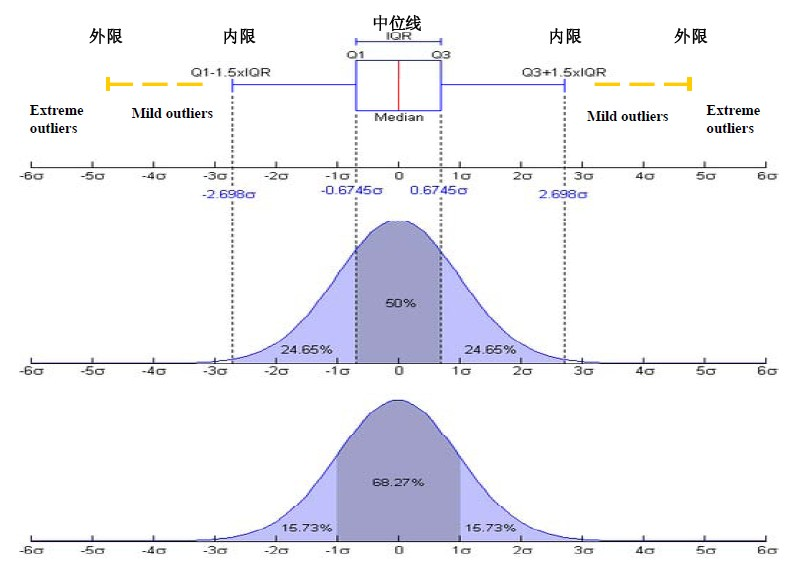• 但是，OPTICS的异常值检测能力受到多个因素限制，例如不同参数和不同点集形状。 因此，提出了一基于OPTICS离群值检测方法，称为OD-OPTICS，它增加了预处理过程并修改了CD计算方法。 首先，执行提供关键...
• 箱线图（Boxplot）也称箱须图（Box-whisker Plot），是利用数据中五个统计量：最小值、第一四分位数、中位数、第四分位数与最大来描述数据种方法，它也可以粗略地看出数据是否具有有对称性，分布分散...
先介绍使用到的方法原理，也就是一种异常检测的方法。
首先要先了解箱线图。

箱线图

箱线图（Boxplot）也称箱须图（Box-whisker Plot），是利用数据中的五个统计量：最小值、第一四分位数、中位数、第三四分位数与最大值来描述数据的一种方法，它也可以粗略地看出数据是否具有有对称性，分布的分散程度等信息，特别可以用于对几个样本的比较。 ——MBAlib 箱线图

先看一下什么是箱线图，下面这个是常见的箱线图样子。具体含义如下，首先计算出第一四分位数（Q1）、中位数、第三四分位数（Q3）。
中位数我们都知道，就是将一组数字按从小到大的顺序排序后，处于中间位置（也就是50%位置）的数字。
同理，第一四分位数、第三四分位数是按从小到大的顺序排序后，处于25%、75%的数字。

令 IQR=Q3−Q1IQR=Q3−Q1 ，那么 Q3+1.5(IQR)Q3+1.5(IQR) 和 Q1−1.5(IQR)Q1−1.5(IQR) 之间的值就是可接受范围内的数值，这两个值之外的数认为是异常值。

在Q3＋1.5IQR（四分位距）和Q1-1.5IQR处画两条与中位线一样的线段，这两条线段为异常值截断点，称其为内限；在Q3＋3IQR和Q1－3IQR处画两条线段，称其为外限。 处于内限以外位置的点表示的数据都是异常值，其中在内限与外限之间的异常值为温和的异常值（mild outliers），在外限以外的为极端的异常值(li)的异常值extreme outliers。这种异常值的检测方法叫做Tukey’s method。

从矩形盒两端边向外各画一条线段直到不是异常值的最远点 表示该批数据正常值的分布区间点，示该批数据正常值的分布区间。
一般用“〇”标出温和的异常值，用“＊”标出极端的异常值。python 代码分享

这段检测异常值的代码是从kaggle上看到的，很简单也很有用。
代码原地址：https://www.kaggle.com/yassineghouzam/titanic-top-4-with-ensemble-modeling/notebook

通过上面对箱线图的介绍，相信同时也清楚了异常值检测的方法。
假设我们现在已经有了一份pandas.DataFrame读取后的数据df，其中需要进行检测的列保存在features列表中，每个样本能忍受的最大异常值数量为n。

# Outlier detection
import pandas as pd
import numpy as np
from collections import Counter

def detect_outliers(df,n,features):

outlier_indices = []

# iterate over features(columns)
for col in features:
# 1st quartile (25%)
Q1 = np.percentile(df[col], 25)
# 3rd quartile (75%)
Q3 = np.percentile(df[col],75)
# Interquartile range (IQR)
IQR = Q3 - Q1

# outlier step
outlier_step = 1.5 * IQR

# Determine a list of indices of outliers for feature col
outlier_list_col = df[(df[col] < Q1 - outlier_step) | (df[col] > Q3 + outlier_step )].index

# append the found outlier indices for col to the list of outlier indices
outlier_indices.extend(outlier_list_col)

# select observations containing more than 2 outliers
outlier_indices = Counter(outlier_indices)
multiple_outliers = list( k for k, v in outlier_indices.items() if v > n )

return multiple_outliers   

# detect outliers from "Col1","Col2","Col3","Col4"
Outliers_to_drop = detect_outliers(df,2,["Col1","Col2","Col3","Col4"])
# Drop outliers
df = df.drop(Outliers_to_drop, axis = 0).reset_index(drop=True)
展开全文• 建立变异模型的方法三种，这里只说两种standard，direct 先新建一个模型 create_variation_model (Width, Height, ‘byte’, ‘standard’, ModelID_Variation) 1.standard 这里如果选择是standard属性那么就是...
建立变异模型的方法有三种，这里只说两种standard，direct
先新建一个模型
create_variation_model (Width, Height, ‘byte’, ‘standard’, ModelID_Variation)
1.standard
这里如果选择的是standard属性那么就是通过train_variation_model来训练多张图像，以获取一个平均的变异图像，再通过prepare_variation_model来建立变异模型。
2.direct
如果选择的是direct属性那么就是通过sobel_amp来用一张OK的图像直接生成一个变异图像,
再通过prepare_direct_variation_model来建立一个变异模型
简介：
Variation Model的主要原理是将待检测的图像与一张标准图像作比较，找出待检测图像与标准图像（ideal image）的明显差异（也就是不良）。标准图像可以采用几张OK品的图像训练（training）得到，也可以通过对一张OK品图像进行处理得到。训练后得到标准图像和一张variation图像（variation image），variation图像中包含了图像中每个像素点灰度值允许变化的范围。标准图像和variation图像用来创建一个variation model，如此，其他图像就可以与variation model作比较了。
Variation Model方法中常用的算子：
create_variation_model( : : Width, Height, Type, Mode : ModelID)
创建一个ID为ModelID，宽为Width,高为Height,类型为Type的Variation Model,参数Mode决定了创建标准图像和相应的variation图像的方法。'standard’表示标准的训练方法，标准图像的位置是各训练图像位置的平均，'robust’表示鲁棒的训练方法，标准图像的位置是各训练图像的中值，此模式在训练图像中可能存在ERROR时使用，'direct’表示标准图像由单张图像经过处理得到，由此方法得到的标准图像只能应用prepare_direct_variation_model算子得到variation model。
train_variation_model(Images : : ModelID : )训练一个Variation Model。
get_variation_model( : Image, VarImage : ModelID : )返回variation model中的标准图像（Image）和variation image（VarImage），此算子主要用来检视创建的variation model是否OK。
prepare_variation_model( : : ModelID, AbsThreshold, VarThreshold : )设置variation model的绝对阈值和相对阈值。绝对阈值即待检测图像与标准图像的差值，相对阈值即待检测图像与variation model与VarThreshold乘绩的差值。
clear_train_data_variation_model( : : ModelID : )清除variation model的训练数据所占用的内存。
compare_variation_model(Image : Region : ModelID : )待检测图像与variation model进行比较，超过阈值的区域在Rgion参数中返回。同threshold一样，返回的区域被看做一个区域，可以使用connection算子进行连通性分析，然后根据区域的特征（如面积）对区域进行选择。
clear_variation_model( : : ModelID : )释放一个variation model的内存空间
PS：在model训练和比较的时候，常常需要对图像进行模板匹配，以使图像准确对齐。
compare_ext_variation_model (ImageScaled, RegionDifference, ModelID_Variation, ‘light_dark’)将图像与变异模型进行比较。获取检测图像和标准图像之间的差异的区域。
总结：
Variation Model使用标准图像与待检测图像灰度值相比较，来判断产品是否OK，适用于印刷品检测及产品表面检测。从实际算法过程可以看出，此检测实际可分为两部分，对于图像中的大面积灰度一致区域，主要利用待检测图像与标准图像（ideal image)比较得出差异区域，对于图像中的边缘位置（edges）区域，主要利用待检测图像与Variation图像（variation image）比较得出差异区域。所以在实际应用中，应根据实际情况设置AbsThreshold和VarThreshold的值。
参考halcon例程


展开全文• 1. 引言 真实数据集中不同维度的数据通常具有高度的相关性，这是因为不同的属性往往是由相同的基础过程以密切相关的方式产生的。在古典统计学中，这被称为...线性相关假设是使用两模型进行异常检测的重要理论基础。
1. 引言
真实数据集中不同维度的数据通常具有高度的相关性，这是因为不同的属性往往是由相同的基础过程以密切相关的方式产生的。在古典统计学中，这被称为——回归建模，一种参数化的相关性分析。
一类相关性分析试图通过其他变量预测单独的属性值，另一类方法用一些潜在变量来代表整个数据。前者的代表是 线性回归，后者一个典型的例子是 主成分分析。本文将会用这两种典型的线性相关分析方法进行异常检测。
需要明确的是，这里有两个重要的假设：
假设一：近似线性相关假设。线性相关假设是使用两种模型进行异常检测的重要理论基础。
假设二：子空间假设。子空间假设认为数据是镶嵌在低维子空间中的，线性方法的目的是找到合适的低维子空间使得异常点(o)在其中区别于正常点(n)。
基于这两点假设，在异常检测的第一阶段，为了确定特定的模型是否适合特定的数据集，对数据进行探索性和可视化分析是非常关键的。
2. 数据可视化
#coding:utf-8
#导入warnings包，利用过滤器来实现忽略警告语句。
import warnings
warnings.filterwarnings('ignore')

import pandas as pd
import numpy as np
import matplotlib.pyplot as plt
import seaborn as sns
import missingno as msno

2.1 载入训练集和测试集
path = 'dataverse_files/'

Train_data.head()2.3 通过describe()来熟悉数据的相关统计量
Train_data.describe()2.4 通过info()来熟悉数据类型
Train_data.info()2.5 相关性分析
numeric_features = ['f' + str(i) for i in range(30)]

numeric = Train_data[numeric_features]
correlation = numeric.corr()

f , ax = plt.subplots(figsize = (14, 14))

sns.heatmap(correlation,square = True)
plt.title('Correlation of Numeric Features with Price',y=1,size=16)
plt.show())2.6 每个数字特征的分布可视化
## 3) 每个数字特征的分布可视化
f = pd.melt(Train_data, value_vars=numeric_features)
g = sns.FacetGrid(f, col="variable",  col_wrap=6, sharex=False, sharey=False)
g = g.map(sns.distplot, "value", hist=False, rug=True)2.7 变量两两之间的相关性
sns.set()
sns.pairplot(Train_data[numeric_features],size = 2 ,kind ='scatter',diag_kind='kde')
plt.savefig('correlation.png')
plt.show()2.8 数据降维可视化
from sklearn.manifold import
tsne = TSNE(n_components=2, init='pca', random_state=0)
result = tsne.fit_transform(numeric)
x_min, x_max = np.min(result, 0), np.max(result, 0)
result = (result - x_min) / (x_max - x_min)
label = Train_data['label']
fig = plt.figure(figsize = (7, 7))
#f , ax = plt.subplots()
color = {'o':0, 'n':7}
for i in range(result.shape):
plt.text(result[i, 0], result[i, 1], str(label[i]),
color=plt.cm.Set1(color[label[i]] / 10.),
fontdict={'weight': 'bold', 'size': 9})
plt.xticks([])
plt.yticks([])
plt.title('Visualization of data dimension reduction')3. 线性回归
在线性回归中，我们假设不同维度的变量具有一定的相关性，并可以通过一个相关系数矩阵进行衡量。因此对于特定的观测值，可以通过线性方程组来建模。在实际应用中，观测值的数量往往远大于数据的维度，导致线性方程组是一个超定方程，不能直接求解。因此需要通过优化的方法，最小化模型预测值与真实数据点的误差。
线性回归是统计学中一个重要的应用，这个重要的应用往往是指通过一系列自变量去预测一个特殊因变量的值。在这种情况下，异常值是根据其他自变量对因变量的影响来定义的，而自变量之间相互关系中的异常则不那么重要。这里的异常点检测主要用于数据降噪，避免异常点的出现对模型性能的影响，因而这里关注的兴趣点主要是正常值(n)。
而我们通常所说的异常检测中并不会对任何变量给与特殊对待，异常值的定义是基于基础数据点的整体分布，这里我们关注的兴趣点主要是异常值(o)。
广义的回归建模只是一种工具，这种工具既可以用来进行数据降噪也可以进行异常点检测。
3.1 基于自变量与因变量的线性回归
3.1.1 最小二乘法
为了简单起见，这里我们一元线性回归为例:
$Y=\sum_{i=1}^{d} a_{i} \cdot X_{i}+a_{d+1}$
变量Y为因变量，也就是我们要预测的值；$X_{1}...X_{d}$为一系列因变量，也就是输入值。系数$a_{1}...a_{d+1}$为要学习的参数。假设数据共包含$N$个样本，第$j$个样本包含的数据为$x_{j1}...x_{jd}$和$y_{j}$，带入式(1)如下式所示：
$y_{j}=\sum_{i=1}^{d} a_{i} \cdot x_{j i}+a_{d+1}+\epsilon_{j}$
这里$\epsilon_{j}$为第$j$个样本的误差。以$Y$ 代表 $N \times 1$ 的因变量矩阵${(y_{1}...y_{N})}^{T}$，即样本中的真实值；以$U$代表$N \times (d+1)$的自变量矩阵，其中第$j$行为$(x_{j1}...x_{jd}, 1)$；以$A$ 代表 $(d+1) \times 1$ 的系数矩阵$(a_{1}...a_{d+1})^{T}$。则模型可表示为： $f(U, A) = U \cdot A$
定义目标函数为：
$L(A) = \frac{1}{2}{\left| {Y - U \cdot A} \right|^2}$
目标函数是关于$A$的凸函数，其对$A$求偏导为：
$\frac{{\partial L(A)}}{{\partial A}} = \frac{1}{2}\frac{{\partial {{\left| {Y - U \cdot A} \right|}^2}}}{{\partial A}} = - {U^T}(Y - U \cdot A)$
令$\frac{{\partial L(A)}}{{\partial A}}=0$，得到最优参数为：
$A=\left(U^{T} \cdot U\right)^{-1} \cdot\left(U^{T} \cdot Y\right)$
这种求解线性回归参数的方法也叫最小二乘法。
最小二乘法要求矩阵 $U^{T} \cdot U$ 可逆，即$U^{T} \cdot U$是满秩的。当$U^{T} \cdot U$不可逆时可以通过两种方法进行参数估计，一种先使用主成分分析等方法来预处理数据，消除不同特征之间的相关性，然后再使用最小二乘法。第二种方法是使用梯度下降法。
3.1.2 梯度下降法
数据集
监督学习一般靠数据驱动。我们通常收集一系列的真实数据，例如多栋房屋的真实售出价格和它们对应的面积和房龄。我们希望在这个数据上面寻找模型参数来使模型的预测价格与真实价格的误差最小。在机器学习术语里，该数据集被称为训练数据集（training data set）或训练集（training set），通常还应该有一个用于防止过拟合的交叉验证集和一个用于评估模型性能的测试集(test set)。一栋房屋被称为一个样本（sample），其真实售出价格叫作标签（label），用来预测标签的两个因素叫作特征（feature）。
损失函数
如果把线性回归看作是一个优化问题，那么我们要优化的目标就是损失函数。损失函数是用来衡量样本误差的函数，我们的优化目标是要求得在误差最小的情况下模型参数的值。这里强调一下损失函数和代价函数的区别：

注意： Loss Function(损失函数)：the error for single training example; **Cost Function(代价函数)：**the average of the loss functions of the entire training set;

线性回归常用的损失函数是均方误差，表达式为：
$l^{(i)}(\mathbf{w}, b)=\frac{1}{2}\left(\hat{y}^{(i)}-y^{(i)}\right)^{2}$
$L(\mathbf{w}, b)=\frac{1}{n} \sum_{i=1}^{n} l^{(i)}(\mathbf{w}, b)=\frac{1}{n} \sum_{i=1}^{n} \frac{1}{2}\left(\mathbf{w}^{\top} \mathbf{x}^{(i)}+b-y^{(i)}\right)^{2}$   其中 $\hat{y}$ 为预测值，$y$ 为真实值。 优化算法 - 随机梯度下降
当模型和损失函数形式较为简单时，上面的误差最小化问题的解可以直接用公式表达出来。这类解叫作解析解（analytical solution）。本节使用的线性回归和平方误差刚好属于这个范畴。然而，大多数深度学习模型并没有解析解，只能通过优化算法有限次迭代模型参数来尽可能降低损失函数的值。这类解叫作数值解（numerical solution）。
$(\mathbf{w}, b) \leftarrow(\mathbf{w}, b)-\frac{\eta}{|\mathcal{B}|} \sum_{i \in \mathcal{B}} \partial_{(\mathbf{w}, b)} l^{(i)}(\mathbf{w}, b)$
学习率($\eta$): 代表在每次优化中，能够学习的步长的大小 批量大小($B$): 是小批量计算中的批量大小batch size
3.2 基于异常检测的线性回归
前一节讨论了这样一种情况：即一个特定的变量被认为是特殊的，最优平面是通过最小化该特殊变量的均方误差而确定的。而我们通常所说的异常检测中并不会对任何变量给与特殊对待，异常值的定义是基于基础数据点的整体分布，因此需要采用一种更一般的回归建模：即以相似的方式对待所有变量，通过最小化数据对该平面的投影误差确定最佳回归平面。在这种情况下，假设我们有一组变量 $X_{1}… X_{d}$， 对应的回归平面如下：
$a_{1} \cdot X_{1}+\ldots+a_{d} \cdot X_{d}+a_{d+1}=0$
为了后续计算的方便，对参数进行如下约束： $\sum\limits_{i = 1}^d {a_i^2 = 1}$   以$L_{2}$范数作为目标函数： $L = {\left| {U \cdot A} \right|_2}$
这样的一个问题可以通过主成分分析方法得到有效解决，我们会单独用一个部分进行讨论。
4、主成分分析
上一节的最小二乘法试图找到一个与数据具有最佳匹配 $(d−1)$ 维超平面。主成分分析方法可用于解决这一问题的广义版本。具体来说，它可以找到任意 $k( k 维的最优表示超平面，从而使平方投影误差最小化。
4.1 原理推导
对于 $d$ 维，包含 $N$ 个样本的数据，用 $R_{i}$ 表示其中第 $i$ 行为：$[x_{i1}... x_{id}]$。由此可以得到 $d \times d$ 的协方差矩阵（标准的PCA应当计算相关系数矩阵，即对数据进行均值为0方差为1的标准化处理，而协方差矩阵只需要减去均值即可）:
$Σ = (R - \bar{R})^{T} \cdot (R - \bar{R})$
易知协方差矩阵 $Σ$ 是对称并且半正定的，因此可以进行相似对角化：
$Σ = P \cdot D \cdot P^{T}$
这里的 $D$ 为对角矩阵，对角元素为特征值；$P$ 为标准正交矩阵，每一行为对应的特征向量；这些标准正交向量提供了数据应该投影的轴线方向。与异常检测相关的主成分分析的主要性质如下：
如果前 $k$ 的特征向量选定之后（根据最大的$k$个特征值），由这些特征向量定义的 $k$ 维超平面是在所有维度为 $k$ 的超平面中，所有数据点到它的均方距离尽可能小的平面。
如果将数据转换为与正交特征向量对应的轴系，则转换后的数据沿每个特征向量维的方差等于相应的特征值。在这种新表示中，转换后的数据的协方差为0。
由于沿特征值小的特征向量的转换数据的方差很低，因此沿这些方向的变换数据与平均值的显着偏差可能表示离群值。
需要注意的是，相比2.2节的内容，这里提供了一个更加普遍的解决方法。2.2中的内容可以归为主成分分析中只保留最大特征值对应的特征向量的情况。
在得到这些特征值和特征向量之后，可以将数据转换到新的坐标系中。以 $Y_{1}...Y_{N}$ 表示新坐标系中的数据，这些数据可以通过原始向量 $R_{i}$ 与包含新轴系的标准正交特征向量矩阵 $P$ 的乘积来实现。 ${Y_i} = {R_i} \cdot P$
在许多涉及高维数据集的真实场景中，很大一部分特征值往往非常接近于零。这意味着大多数数据都沿着一个低维的子空间排列。从异常检测的角度来看，这是非常方便的，因为离这些投影方向非常远的观测值可以被假定为离群值。例如，对于特征值较小（方差较小）的特征向量 $j$，第 $i$ 条记录的 $y_{ij}$ 与 $y_{kj}$ 的其他值的偏差较大，说明有离群行为。这是因为当$j$固定而$k$变化时，$y_{kj}$ 的值应当变化不大。因此，$y_{ij}$ 值是不常见的。
在不选取任何特定的 $k$ 维集合的情况下，一种更精确的异常检测建模方法是使用特征值来计算数据点沿每个主分量方向到质心的归一化距离。设 $e_{j}$为第 $j$ 个特征向量，$λ_{j}$ 为沿该方向的方差(特征值)。数据点$\bar{X}$相对于对数据质心$\bar{\mu}$的总体归一化异常得分可以由下式给出:
$S \operatorname{core}(\bar{X})=\sum_{j=1}^{d} \frac{\left|(\bar{X}-\bar{\mu}) \cdot \bar{e}{j}\right|^{2}}{\lambda{j}}$
值得注意的是，对异常得分的大部分贡献是由 $λ_{j}$ 值较小的主成分的偏差提供的，这一点上文中有提及过。主成分分析比因变量回归能更稳定地处理少数异常值的存在。这是因为主成分分析是根据最优超平面来计算误差的，而不是一个特定的变量。当数据中加入更多的离群点时，最优超平面的变化通常不会大到影响离群点的选择。因此，这种方法更有可能选择正确的异常值，因为回归模型一开始就更准确。
4.2 归一化问题
当不同维度的尺度差别较大时，使用 $PCA$ 有时并不能得到直观有效的结果。例如，考虑一个包含年龄和工资等属性的人口统计数据集。工资属性的范围可能是几万，而年龄属性几乎总是小于100，使用主成分分析会导致主成分被高方差属性所控制。对于一个只包含年龄和工资的二维数据集，最大的特征向量几乎与工资轴平行，这会降低异常点检测过程的有效性。因此，一个自然的解决方案是对数据进行均值为0方差为1的标准化处理。这隐含地导致在主成分分析中使用相关矩阵而不是协方差矩阵。当然，这个问题并不是线性建模所独有的，对于大多数异常检测算法，都需要使用这样的预处理。
5、回归分析的局限性
回归分析作为检测离群值的工具有一些局限性。这些缺点中最重要的是在本章的一开始就讨论了，其中探讨了回归分析的数据特定性质。特别是，为了使回归分析技术有效，数据需要高度相关，并沿着低维子空间对齐。当数据不相关，但在某些区域高度聚集时，这种方法可能不会有效。
另一个相关的问题是，数据中的相关性在本质上可能不是全局性的。最近的一些分析观察表明，子空间相关性是特定于数据的特定位置的。在这种情况下，由主成分分析发现的全局子空间对于异常检测是次优的。因此，为了创建更一般的局部子空间模型，有时将线性模型与邻近模型(在后续章节中讨论)结合起来是有用的。这将是高维和子空间异常检测的主题，将在后续章节详细讨论。
6、总结
真实数据中，数据不同属性之间往往具有显著的相关性。在这种情况下，线性建模可以提供一种有效的工具来从底层数据中移除异常值或者进行异常检测。对于其他基于因变量回归的应用，线性建模是一种工具，去除异常值对于提高此类应用的性能是非常重要的。在大多数情况下，主成分分析提供了去除异常值和进行异常检测最有效的方法，因为它对存在少数异常值的数据更有鲁棒性。
7.资料
数据集
8、练习
from __future__ import division
from __future__ import print_function

import os
import sys

from pyod.models.pca import PCA
from pyod.utils.data import generate_data
from pyod.utils.data import evaluate_print
from pyod.utils.example import visualize

if __name__ == "__main__":
contamination = 0.1  # percentage of outliers
n_train = 200  # number of training points
n_test = 100  # number of testing points

# Generate sample data
X_train, y_train, X_test, y_test = \
generate_data(n_train=n_train,
n_test=n_test,
n_features=20,
contamination=contamination,
random_state=42)

# train PCA detector
clf_name = 'PCA'
clf = PCA(n_components=3)
clf.fit(X_train)

# get the prediction labels and outlier scores of the training data
y_train_pred = clf.labels_  # binary labels (0: inliers, 1: outliers)
y_train_scores = clf.decision_scores_  # raw outlier scores

# get the prediction on the test data
y_test_pred = clf.predict(X_test)  # outlier labels (0 or 1)
y_test_scores = clf.decision_function(X_test)  # outlier scores

# evaluate and print the results
print("\nOn Training Data:")
evaluate_print(clf_name, y_train, y_train_scores)
print("\nOn Test Data:")
evaluate_print(clf_name, y_test, y_test_scores)展开全文python 数据挖掘
• 经典计算数据集中疑似异常值的方法是Tukey method。该方法先计算出数据集四分之一分位数（Q1）和四分之分位数（Q3），从而计算出四分位数间距（IQR），然后将小于Q1 - 1.5IQR或者大...
在数据集中如果某一个观察值不寻常地大于或者小于该数据集中的其他数据，我们则称之为疑似异常值。疑似异常值的存在，会对随后的计算结果产生不适当的影响，检测疑似异常值并加以适当的处理是十分必要的。
一种经典的计算数据集中疑似异常值的方法是Tukey method。该方法先计算出数据集的四分之一分位数（Q1）和四分之三分位数（Q3），从而计算出四分位数间距（IQR），然后将小于Q1 - 1.5IQR或者大于Q3 + 1.5IQR的数据点当做是疑似异常值。我们可以借助这种方法在DataFrame中检测异常值。代码如下：
import numpy as np
from collections import Counter

# Outlier detection
def detect_outliers(df, n, features):
"""
Takes a dataframe df of features and returns a list of the indices
corresponding to the observations containing more than n outliers according
to the Tukey method.
"""
outlier_indices = []

# iterate over features(columns)
for col in features:
# 1st quartile (25%)
Q1 = np.percentile(df[col], 25)
# 3rd quartile (75%)
Q3 = np.percentile(df[col], 75)
# quartile spacing (IQR)
IQR = Q3 - Q1
# outlier step
outlier_step = 1.5 * IQR

# Determine a list of indices of outliers for feature col
outlier_list_col = df[(df[col] < Q1 - outlier_step) | (df[col] > Q3 + outlier_step)].index

# append the found outlier indices for col to the list of outlier indices
outlier_indices.extend(outlier_list_col)

# select observations containing more than n outliers
outlier_indices = Counter(outlier_indices)
multiple_outliers = list(k for k, v in outlier_indices.items() if v > n)

return multiple_outliers

参考
Tukey method
概率论与数理统计 第四版 浙江大学出版社

以上就是本文的全部内容，如果您喜欢这篇文章，欢迎将它分享给朋友们。
感谢您的阅读，祝您生活愉快！
作者：小美哥
2019-02-02


展开全文Pandas
• 异常检测（或异常值检测）是对稀有物品、事件或观察结果识别，这些物品、事件或观察结果与大多数数据存在显著差异，从而引起疑虑。通常，异常数据可以关联到某种问题或罕见事件，例如银行欺诈、医疗问题、结构缺陷...
• 文章目录前言一、基于距离的方法1. 单元格方法2. 索引方法二、基于密度的方法三、LOF方法...该方法基于最近邻距离来定义异常值。 前提假设-------异常点 kkk 近邻距离要远大于正常点。 嵌套循环法------- (1)..数据分析
• 、线性模型 1、引言 真实数据集中不同维度的数据通常具有高度的相关性，这是因为不同的属性往往是由相同的基础过程以密切相关的方式产生的。...线性相关假设是使用两模型进行异常检测的重要理论算法 python 机器学习 人工智能
• 目录 异常值 异常值的三种主要类型 点异常值 上下文异常值 集体异常值 ...异常值检测方法 ...使用机器学习DBSCA和PCA法----》基于密度的聚类方法和主成分分析 ...单变量异常值检测方法：T图...单变量异常值检测方法：T图基箱python 数据分析 人工智能 机器学习
• 在中等高维数据集上执行异常值检测的另一有效方法是使用局部异常因子（Local Outlier Factor ，LOF）算法。1、算法思想LOF通过计算一个数值score来反映一个样本的异常程度。这个数值的大致意思是：一个样本点周围...
• 文章目录引言一、线性回归二、梯度下降法、基于异常检测的线性回归四、归一化问题五、回归分析的局限性总结 引言 真实数据集中不同维度的数据通常具有高度的相关性，这是因为不同的属性往往是由相同的基础过程以...python
• 1，针对监控视频中的异常行为尤其是暴力打斗行为难以准确快速发现问题，提出了一基于维卷积深度网络暴力检测方法。该方法利用大量带标签视频数握进行有监督学习，通过将传统二维卷积核扩展为维来提取...
• 对于结果评价一般大家熟悉Z比分直，En外，我们下面介绍三种另外评价方法，即格鲁布斯(Grubbs)检验、F检验、t检验。今天我们分别来介绍一下。 格鲁布斯(Grubbs)检验 格鲁布斯检验是离散检验一种，...
• 提出了一基于水平样条分割野外导航路径提取方法。 首先，通过OTSU阈值算法对RGB color.space中彩色图像进行预处理，以分割犁沟图像。棉田图像成分分为四类：犁沟（成分包括土地，枯萎叶子等）。 。）...
• 使用三角形表现电压负荷，通过校正功率因数，分析用电异常情况，在每个负荷导纳上并联一个补偿电纳计算出动态平衡电压需要补偿电压，利用差分阈值将不平衡电压中负向电压转换成正向电压，采用大数据分析法对...
• 背景：脑电图（EEG）被定义为一种检测表示脑电导率脑电波的方法。 在全球范围内，脑电图用于进一步分类神经精神疾病。 目的：评估异常脑电图和相关神经系统疾病患病率及其与年龄和性别关系。 方法：于2018年6...
• Go 语言提供了一机制在运行时更新变量和检查它们的值、调用它们的方法，但是在编译时并不知道这些变量具体类型，这称为反射机制。 2. 反射作用 1.在编写不定传参类型函数时候，或传入类型过多时 典型应用是...go go语言
• 三种测试方法：功能测试，边界测试和负面测试(考察各种错误输入)。 在写函数时，如果要检测调用接口是否成功，最好是有一个整数返回值，这样才能确定，调用接口是否成功。 可以在出问题时抛出一个异常。 题目 数值...
• select方法是多个UNIX平台支持的非常常见的I/O多路复用技术，通过描述符集合来表示检测的I/O对象，通过个不同的描述符集合来描述检测I/O事件，可读，可写，异常 。 select 的缺点是 所支持的文件描述符的个数是...
• 开启简约模式的方法是：打开程序所在目录下“Settings.ini”文件（如果没有可以自己创建），将其中“FormStyle”一项的值改为“Simple”并保存即可。 程序有高级筛选功能，开启该功能后用户可以自主选择要修复...
• 种方法： 机器安装就绪后 （1）开机时按住电源键等待机器发出声音（1到2声）后放开； （2）先按住进纸键再按电源键开机，3秒后再放开进纸键。 1、BJC265SP、4310、4650：上纸后按POWER键听一声，等待自检； 2...
• 单一异常值检测 shidetify模块将单个异常检测方法应用于数据。 应用此方法时需要小心。 实际上，通常存在数据可能受到沼泽和掩盖不利影响影响风险。 除非您确定自己在做什么，否则建议您使用mhidetify模块。 ...C++
• 什么是Optional Optional 是个容器：它可以保存类型T的值，或者仅仅...创建Optional 三种方法 //创建一个一定是非空值Optional容器 Optional<Car> emptyCar = Optional.empty(); //创建一个一定是非空值..
• 数据质量分析是数据挖掘中数据准备过程重要一环，是数据预处理前提。数据质量分析主要任务是检查原始数据中是否存在脏...异常值就是不合理数据，常用的检测方法如下： 1） 简单最大最小统计，比如用户...数据挖掘 数据质量分析
• 5.1.1 了解Spring 数据访问异常体系 5.1.2 数据访问模板化 5.1.3 使用DAO 支持类 5.2 配置数据源 5.2.1 使用JNDI 数据源 5.2.2 使用数据源连接池 5.2.3 基于JDBC驱动数据源 5.3 在Spring 中使用JDBC ...
• 开启简约模式的方法是：打开程序所在目录下“Settings.ini”文件（如果没有可以自己创建），将其中“FormStyle”一项的值改为“Simple”并保存即可。 程序有高级筛选功能，开启该功能后用户可以自主选择要修复...
• 开启简约模式的方法是：打开程序所在目录下“Settings.ini”文件（如果没有可以自己创建），将其中“FormStyle”一项的值改为“Simple”并保存即可。 程序有高级筛选功能，开启该功能后用户可以自主选择要修复...
• 01他做了什么事新方法可以实时自动检测和控制异常值和时钟跳变。此外，对时钟模型参数(包括时钟偏移，时钟速度和时钟漂移)进行分类，以确定新模型中自适应因素。(Cui和Yang 2006；Yang和Cui 2008)因此，可以更有效......